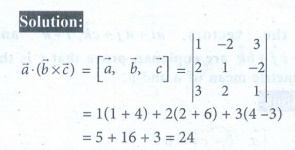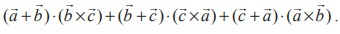Home | | Maths 12th Std | Exercise 6.2: Scalar triple product

# Exercise 6.2: Scalar triple product

Maths Book back answers and solution for Exercise questions - Mathematics : Vector Algebra: Scalar triple product: Exercise Questions with Answer, Solution

EXERCISE 6.2

1.2. Find the volume of the parallelepiped whose coterminous edges are represented by the vectors -6iˆ +14ˆ+10kˆ14iˆ - 10ˆ6kˆ and 2iˆ ˆ- 2kˆ .3. The volume of the parallelepiped whose coterminus edges are 7iˆ + λ ˆ 3kˆiˆ + 2 ˆ kˆ3iˆ + 7 ˆ+ 5kˆ is 90 cubic units. Find the value of λ .4. If,are three non-coplanar vectors represented by concurrent edges of a parallelepiped of volume 4 cubic units, find the value of5. Find the altitude of a parallelepiped determined by the vectors= 2+ 5 + 3k= + 3  2k  and= -3+  2 if the base is taken as the parallelogram determined byand.6. Determine whether the three vectors 2iˆ + 3 ˆ+ kˆiˆ  2 ˆ+ 2kˆ and 3iˆ + ˆ+ 3kˆ are coplanar.7. Let= ˆ+ ˆ+ ˆk= and = c1ˆ+ cˆ+ c3ˆ. If c= 1 and c= 2 , find csuch that,andare coplanar.8. Ifshow that [] depends on neither x nor y .9. If the vectors are aiˆ ˆ+ akˆ , iˆ kˆ  and ciˆ ˆ+ bkˆ  are coplanar, prove that is the geometric  mean of and .10. Let,be three non-zero vectors such thatis a unit vector perpendicular to bothand. If the angle betweenandis π/6. Show that1. 24

2. 720 cubic units

3. −5

4. ±12

5. 2√3 / 5

6. coplanar

7. 2

Tags : Problem Questions with Answer, Solution , 12th Mathematics : UNIT 6 : Applications of Vector Algebra
Study Material, Lecturing Notes, Assignment, Reference, Wiki description explanation, brief detail
12th Mathematics : UNIT 6 : Applications of Vector Algebra : Exercise 6.2: Scalar triple product | Problem Questions with Answer, Solution